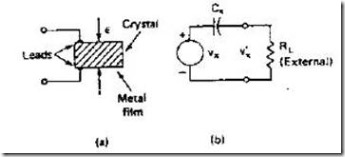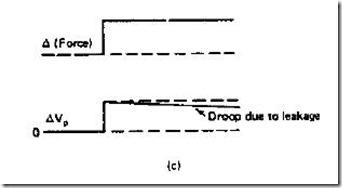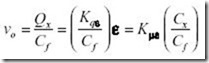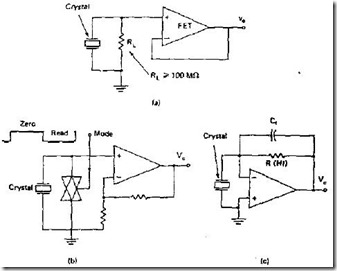" /> Piezoelectric Transducers | TN Industri
Home > English > Piezoelectric Transducers

# Piezoelectric Transducers

Piezoelectric transducers work out the stresses that are formed when the crystals are compressed
• Positive and negative ions are separated by asymmetric crystal structure
• crystalline materials: quartz and barium titanate, polyatidin fluoride electrons
• response formFigure 3.37 Piezoelectric Transducers: (a) PE construction,

(b) PE equivalent circuitFigure 3.38 Voltage Response PE

Series of voltage readers on piezoelectric sensors
• non-conductor crystal (do not measure DC, equivalent circuit) use Op-Amp circuit with high input impedance (FET, for low frequency)
• when the measured response is close to the crystal resonance frequency, measure the charge in place of the voltagewhere Q x = the electrical charge of the crystal (coulomb)
K qe = crystal constants (coul / cm)
ε = compression force (Newton)

• Figure (a) The high R for the DC groove, (b) the switch to measure the strain voltage when ON and OFF and (c) measure the charge, the resulting voltage ( V o ) is:Figure 3.39 Crystal crystal reading circuit